## Split dimensions into two parts in Excel

This article explains how to use formula to split dimensions in a cell into two parts without units (individual length and width).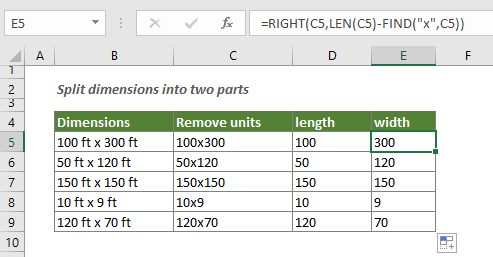#### How to split dimensions into two parts?

You need to apply three formulas separately to split dimensions into individual length and width without units.

Generic formula of removing units from dimension

=SUBSTITUTE(SUBSTITUTE(text,"units","")," ","")

Generic formula of extracting the first dimension

=LEFT(text,FIND("char",text)-1)

Generic formula of extracting the second dimension

=RIGHT(text,LEN(text)-FIND("char",text))

Arguments of above formulas

Text: The string you want to extract the given number of characters from it. It can be:

• 1. The text enclosed in quotation marks;
• 2. Or a reference to a cell containing the text.

Units: The unit in the cell that you want to remove.

Char: The character you will split the dimensions based on.

How to use these formulas?

Supposing you have a dimensions list as the below screenshot shown, please do as follows step by step to split it into two parts without units.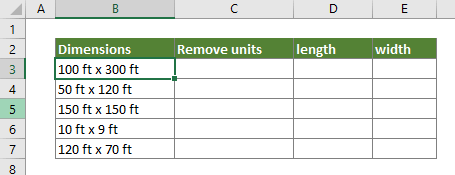##### Firstly, you need to remove the units from the dimensions

1. Select a blank cell to output the dimensions without units.

2. Enter the below formula into it and press the Enter key. Select the result cell and then drag the Fill Handle to apply the formula to other cells as you need.

=SUBSTITUTE(SUBSTITUTE(B3,"ft","")," ","")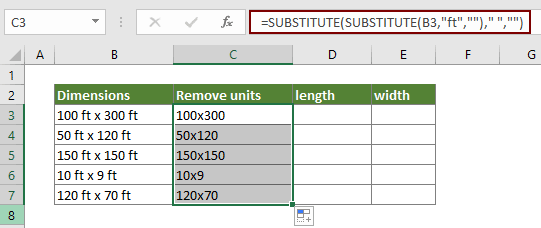##### Secondly, get the first dimension

1. Select a blank cell to place the first dimension.

2. Enter the below formula into it and press the Enter key. Select the result cell and then drag the Fill Handle to apply the formula to other cells as you need.

=LEFT(C3,FIND("x",C3)-1)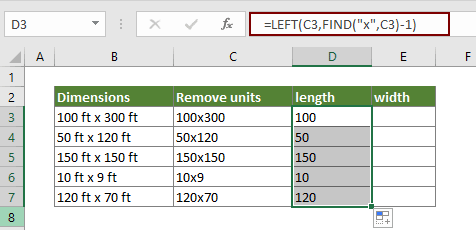##### Finally, get the last dimension

1. Select a blank cell to place the last dimension.

2. Enter the below formula into it and press the Enter key. Select the result cell and then drag the Fill Handle to apply the formula to other cells as you need.

=RIGHT(C3,LEN(C3)-FIND("x",C3))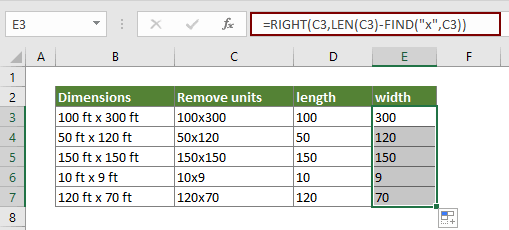How these formulas work?

=SUBSTITUTE(SUBSTITUTE(B3,"ft","")," ","")

• 1. SUBSTITUTE(B3,"ft",""): The inner SUBSTITUTE function removes all "ft" from B3. The result is 100 x 300
• 2. SUBSTITUTE("100 x 300 "," ",""): The outer SUBSTITUTE function removes all spaces from B3 and returns the result as 100x300.

=LEFT(C3,FIND("x",C3)-1)

• 1. FIND("x",C3)-1: The FIND function locates the "x" in C3. For getting the first dimension without the "x", you need to subtract 1. Then the result is 4-1=3.
• 2. LEFT(C3,3): The LEFT function extract 3 characters starting from the left side of C3 and returns the result as 100.

=RIGHT(C3,LEN(C3)-FIND("x",C3))

• 1. LEN(C3): The LEN function calculates the total string length in C3 and returns the result as 7;
• 2. FIND("x",C3): The FIND function locates the "x" in C3. The result is 4;
• 3. RIGHT(C3,7-4): And then the RIGHT function extracts 3 characters starting from the right side of C3 and returns the last dimension as 300.

#### Related functions

Excel LEFT function
The LEFT function extracts the given number of characters from the left side of a supplied string.

Excel RIGHT function
The RIGHT function extracts a specific number of characters from the right side of the text string.

Excel FIND function
The FIND function is used to find a string within another string, and returns the starting position of the string inside another one.

Excel LEN function
The LEN function returns the number of characters in a text string.

Excel SUBSTITUTE function
The Microsoft Excel SUBSTITUTE function replaces text or characters within a text string with another text or characters.

#### Related formulas

Split dimensions into individual length, height and width
This article explains how to split dimensions in a cell into three parts (individual dimensions that include length, height and width).

Separate numbers from units of measurement
This article explains how to use formula to separate numbers from unites of measurement.

### The Best Office Productivity Tools

#### Kutools for Excel - Helps You To Stand Out From Crowd

 Popular Features: Find, Highlight or Identify Duplicates  |  Delete Blank Rows  |  Combine Columns or Cells without Losing Data  |  Round without Formula ... Super VLookup: Multiple Criteria  |  Multiple Value  |  Across Multi-Sheets  |  Fuzzy Lookup... Adv. Drop-down List: Easy Drop Down List  |  Dependent Drop Down List  |  Multi-select Drop Down List... Column Manager: Add a Specific Number of Columns  |  Move Columns  |  Toggle Visibility Status of Hidden Columns  |  Compare Columns to Select Same & Different Cells ... Featured Features: Grid Focus  |  Design View  |  Big Formula Bar  |  Workbook & Sheet Manager | Resource Library (Auto Text)  |  Date Picker  |  Combine Worksheets  |  Encrypt/Decrypt Cells  |  Send Emails by List  |  Super Filter  |  Special Filter (filter bold/italic/strikethrough...) ... Top 15 Toolsets:  12 Text Tools (Add Text, Remove Characters ...)  |  50+ Chart Types (Gantt Chart ...)  |  40+ Practical Formulas (Calculate age based on birthday ...)  |  19 Insertion Tools (Insert QR Code, Insert Picture from Path ...)  |  12 Conversion Tools (Numbers to Words, Currency Conversion ...)  |  7 Merge & Split Tools (Advanced Combine Rows, Split Excel Cells ...)  |  ... and more

Kutools for Excel Boasts Over 300 Features, Ensuring That What You Need is Just A Click Away...#### Office Tab - Enable Tabbed Reading and Editing in Microsoft Office (include Excel)

• One second to switch between dozens of open documents!
• Reduce hundreds of mouse clicks for you every day, say goodbye to mouse hand.
• Increases your productivity by 50% when viewing and editing multiple documents.
• Brings Efficient Tabs to Office (include Excel), Just Like Chrome, Edge and Firefox.